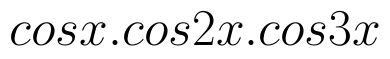Select Page

# CBSE Maths 12 Science Continuity and Differentiability MCQ Solutions in Hindi

CBSE Maths 12 Science Continuity and Differentiability MCQ Solutions in Hindi to enable students to get Solutions in a narrative video format for the specific question.

Expert Teacher provides CBSE Maths 12 Science Continuity and Differentiability MCQ Solutions through Video Solutions in Hindi language. This video solution will be useful for students to understand how to write an answer in exam in order to score more marks. This teacher uses a narrative style for a question from Continuity and Differentiability not only to explain the proper method of answering question, but deriving right answer too.

Please find the question below and view the Solution in a narrative video format.

Question:

Solution Video in Hindi:

You can select video Solutions from other languages also. Please check Solutions in ( English )

## Similar Questions from CBSE, 12th Science, Maths, Continuity and Differentiability

Question 1 : Differentiate the function w.r.t.x. (View Answer Video)

Question 2 :  Find the second order derivative of the function. (View Answer Video)

Question 3 : Differentiate the function w.r.t.x. (View Answer Video)

Question 4 : Differentiate the functionwith respect to x. (View Answer Video)

Question 5 : Differentiate the function w.r.t.x. (View Answer Video)

### Vector Algebra

Question 1 :  Find the sum of the vectors :(View Answer Video)

Question 2 :  Write a vector in the direction of the vectorthat has magnitude 9 units.   (View Answer Video)

Question 3 :  Find the sum of the vectorsand(View Answer Video)

Question 4 : Find a vector in the direction ofthat has magnitude 7 units.   (View Answer Video)

Question 5 :  Write a unit vector in the direction of the sum of vectorsand(View Answer Video)

### Determinant

Question 2 : Evaluate the determinant:. (View Answer Video)
Question 4 : Find the inverse of the matrix. (View Answer Video)
Question 5 : If, then x is equal to, (View Answer Video)
Question 1 :is equal to :